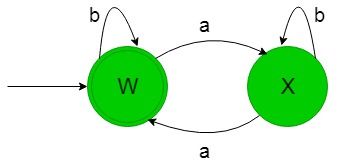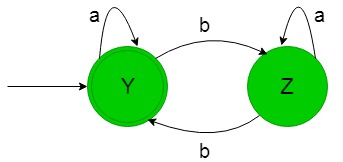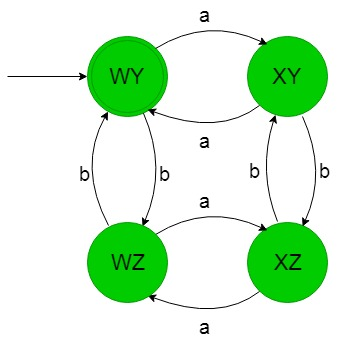TOC | Cross Product Operation in DFA

Prerequisite: Designing finite automata
Let’s understand the cross product operation in Deterministic Finite Automata (DFA) with help of the below example-

Designing a DFA for the set of string over {a, b} such that string of the language contains even number of a’s and b’s then desired language will be like below-

L = {ε, aa, bb, abab, aabb, baba, bbaa, .......}

Let’s see steps for cross product operation in DFA:

Step-1:
Let’s form a DFA which count even number of a’s-
In the below state transition diagram, ‘W’ is the initial state and final state too, which accept language of string containing even number of a’s and any number of b’s.The language accepted by above DFA is-

L = {ε, aab, b, baa, aabbbbb, aaaab, ..........}

The language does not accepted by above DFA is-

L = {aaa, abbb, baaa, bbaaba, ...........}

Step-2:
Let’s form a DFA which count even number of b’s-
In the below state transition diagram, ‘Y’ is the initial state and final state too, which accept language of string containing even number of b’s and any number of a’s.The language accepted by above DFA is-

L = {ε, bba, a, abb, bbbbaaaa, bbbba, ...........}

The language does not accepted by above DFA is-

L = {bbb, bbba, abbb, aaba, ...........}

Step-3:
Here the states of step-1 and step-2 get cross multiplied and produce a result like below-

{W, X} * {Y, Z} = {WY, WZ, XY, XZ}

And in the below, state transition diagram four states {WY, WZ, XY, XZ} used is the result of the cross product of step 1 and 2 states out of which ‘WY’ is the initial and final state too because in step 1 ‘W’ is the initial and final state and in step 2 ‘Y’ is the initial and final state and rest are normal states.
Then the resultant state transition diagram after cross product operation becomes like below-Thus the above DFA accepts all the language of an even number of a’s and b’s string and the language which is accepted and not accepted by above DFA is given below-

L1 = {ε, aa, bb, abab, aabb, baba, bbaa, .......}
L2 = {aaa, aaabb, aaabaabb, aaabb, baaba, bbbaa, .......}

L1 is accepted by above DFA but L2 does not.My Personal Notes arrow_drop_upCheck out this Author's contributed articles.

If you like GeeksforGeeks and would like to contribute, you can also write an article using contribute.geeksforgeeks.org or mail your article to contribute@geeksforgeeks.org. See your article appearing on the GeeksforGeeks main page and help other Geeks.

Please Improve this article if you find anything incorrect by clicking on the "Improve Article" button below.